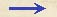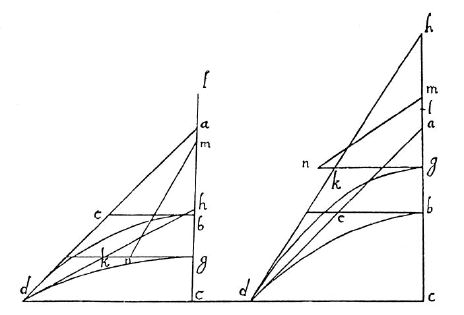Discorsi Propositions 3/07-th-04 Discorsi Proposition3/07-th-04THEOREMA, PROPOSITIO VII. THEOREM. PROPOSITION VIIIn proiectis a quibus semiparabolae eiusdem amplitudines describuntur, {20} minor requiritur impetus in eo quod describit illam cuius amplitudo suae altitudinis est dupla, quam in quolibet alio. If projectiles describe semi-parabolas of the same amplitude, the momentum required to describe that one whose amplitude is double its altitude is less than that required for any other. Sit enim semiparabola bd, cuius amplitudo cd dupla sit altitudinis suae cb, et in axe in sublimi extenso ponatur ba altitudini bc aequalis, et iungatur ad, quae semiparabolam tanget in d et horizontalem be secabit in e, eritque be ipsi bc, seu ba, aequalis: constat, ipsam describi a proiecto, cuius impetus aequabilis horizontalis sit qualis est in b cadentis ex quiete in a, impetus vero naturalis deorsum qualis est venientis in c ex quiete in b; ex quo constat, impetum ex istis compositum, quodque in termino d impingit, esse ut diagonalem ae, potentia nempe ipsis ambobus aequalem.Sit {30} modo quaelibet alia semiparabola gd, cuius amplitudo eadem gd, altitudo {295} vero cg minor vel maior altitudine bc, eamque tangat hd, secans horizontalem per g ductam in punto k; et fiat ut hg ad gk, ita kg ad gl: erit, ex antedemonstratis, altitudo gl, ex qua cadens describet parabolam gd.Inter ab et gl media proportionalis sit gm: erit gm tempus et momentum, sive impetus, in g cadentis ex l (positum enim est, ab esse mensuram temporis et impetus).Sit rursus inter bc, cg media gn, quae erit temporis et impetus mensura cadentis ex g in c.Si igitur iungatur mn, erit ipsa impetus mensura proiecti per parabolam dg, illidentis in termino d: quem quidem impetum, maiorem esse dico impetu proiecti per parabolam bd, cuius {10} quantitas erat ut ae.Quia enim gn posita est media inter bc, cg; est autem bc aequalis be, hoc est kg (est enim unaquaeque subdupla dc); erit ut cg ad gn, ita ng ad gk, et ut cg, seu hg, ad gk, ita quadratum ng ad quadratum gk: ut autem hg ad gk, ita facta est kg ad gl: ergo ut ng ad quadratum gk ita kg ad gl.Sed ut kg ad gl, ita quadratum kg ad quadratum gm; media enim est gm ita kg, gl: ergo tria quadrata ng, kg, gm sunt continue proportionalia, et duo extrema ng, gm simul sumpta, id est quadratum mn, maius quam duplum quadrati kg, cuius quadratum ae duplum est: ergo quadratum mn maius est quadrato ae, et linea mn maior linea ea: quod erat demonstrandum. Let bd be a semi-parabola whose amplitude cd is double its altitude cb; on its axis extended upwards lay off ba equal to its altitude bc. Draw the line ad which will be a tangent to the parabola at d and will cut the horizontal line be at the point e, making be equal to bc and also to ba. (Condition 304a) It is evident that this parabola will be described by a projectile whose uniform horizontal momentum is that which it would acquire at b in falling from rest at a and whose naturally accelerated vertical momentum is that of the body falling to c, from rest at b. (Condition 3/04-pr-01) From this it follows that the momentum at the terminal point d, compounded of these two, is represented by the diagonal ae, whose square is equal to the sum of the squares of the two components. Now let gd be any other parabola whatever having the same amplitude cd, but whose altitude cg is either greater or less than the altitude bc. Let hd be the tangent cutting the horizontal {295} through g at k. Select a point l such that hg:gk = gk:gl.(Condition 3/05-pr-02-cor) Then from a preceding proposition [V], it follows that gl will be the height from which a body must fall in order to describe the parabola gd. Let gm be a mean proportional between ab and gl; (Condition 3/04-pr-01) then gm will [Prop. IV] represent the time and momentum acquired at g by a fall from l; for ab has been assumed as a measure of both momentum. Again let gn be a mean proportional between bc and cg; it will then represent the time and momentum which the body acquires at c in falling from g. (Condition 3/04-pr-01) If now we join m and n, this line mn will represent the momentum at d of the projectile traversing the parabola dg; which momentum is, I say, greater than that of the projectile travelling along the parabola bd whose measure was given by ae. For since gn has been taken as a mean proportional between bc and gc; and since bc is equal to be and also to kg (each of them being the half of dc) it follows that cg:gn = gn:gk, and as cg or (hg) is to gk so is ng^2 to gk^2: but by construction hg:gk = gk:gl. Hence ng^2 : gk^2 = gk:gl.But gk:gl = gk^2: gm^2, since gm is a mean proportional between kg and gl. Therefore the three squares ng, kg, mg form a continued proportion, gn^2 : gk^2 = gk^2 : gm^2 And the sum of the two extremes which is equal to the square of mn is greater than twice the square of gk; but the square of ae is double the square of gk. Hence the square of mn is greater than the square of ae and the length mn is greater than the length ae. Q. E. D.Discorsi Propositions 3/07-th-04 Discorsi Proposition3/07-th-04